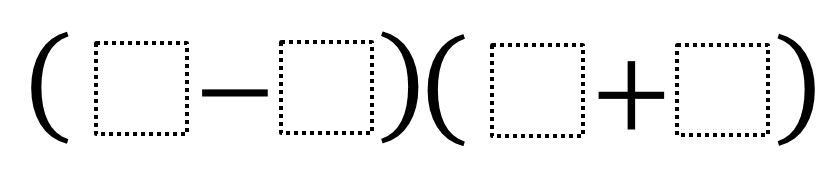Home > Grade 4 > Biggest Product 2

# Biggest Product 2

Directions: Using the numbers 1 through 9, at most one time each, to fill in the boxes and make the biggest/smallest product.### Hint

What numbers should be use to get the biggest product?

biggest: (8+7)(9-1) smallest: (1-2)(8+9)

Source: Nanette Johnson

## Multiplying Differences

Directions: Using the digits 1 to 9, at most one time each, fill in the …

1.Nice exercise in multiplication and flexible thinking, Nanette!

Question: Your answer, smallest: (1-2)(8+9), introduces the possibility of negative numbers. Is that what you intended? If so, I think (1–9)(8+7) is the smallest.

(1–9)(8+7) < (1-2)(8+9)
-48 < -17

If you intended only positive products, then I think that (4–3)(2+1) is the smallest. The 4 and 3 could be replaced by any other consecutive digits between 3 and 9 because their difference will be 1.

2.My student came up with (9 – 8) (1 + 2) = 3 for the smallest answer and (9 – 1) (7 + 8) = 120

3.Mrs. Elliott's 4th graders

We found out that if negative numbers are allowed. The smallest equation would be (1-9)(8+7) which equals -120 not -48.